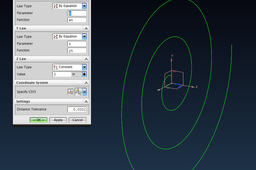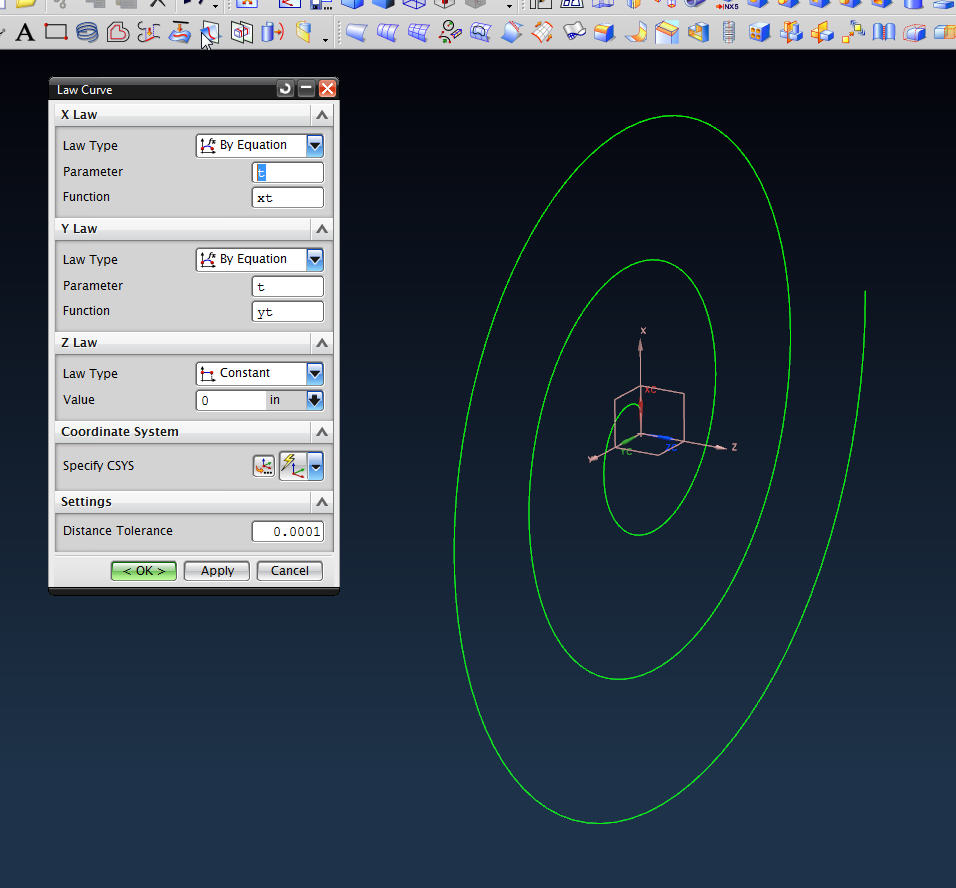# how to draw involute in unigraphics nx 8.5 ?To create a involute curve in NX, you need to use the Law Curve function. But before creating the law curve, you need to define the expressions that control the curve.

1. ### Step 1:

For all law functions you need to define the range over which the formulas are evaluated. NX uses the built in expression "t" as the range and it is evaluated from 0 to 1. You can multiply "t" to get the range desired, i.e., t*5 would cause the function to be evaluated from 0 to 5. So, the first expression you must create is t=1.

2. ### Step 2:

Next you need to create an expression for the number of spirals. Create the expression number_of_turns=3.

3. ### Step 3:

Next create an expression for the beginning radius, r=.5.

4. ### Step 4:

The formulas for the x and y values of an involute are:

x=r*(cos(angle)+(angle*sin(angle)))

y=r*(sin(angle))-(angle*cos(angle)))

where "angle" is the total angle of the involute in radians.

However, NX expects degrees when using trig functions. To get around that you use the number of turns multiplied by 360 degrees for the trig functions and the number of turn multiplied by 2pi elsewhere.

So you expressions for x and y become:

xt=r*((cos(360*t*number_of_turns)+(number_of_turns*t*2*pi())*sin(360*t*number_of_turns)))

and

yt=r*((sin(360*t*number_of_turns)-(number_of_turns*2*t*pi())*cos(360*t*number_of_turns)))

5. ### Step 5:

Once all of these expressions are created, use Insert>Curve>Law Curve to create the involute.

For the X law, select "By Equation", Parameter = t, and Function = xt.

For the Y law, select "By Equation", Parameter = t, and Function = yt.

For the Z law, select "Constant", Value = 0.

Then click OK.